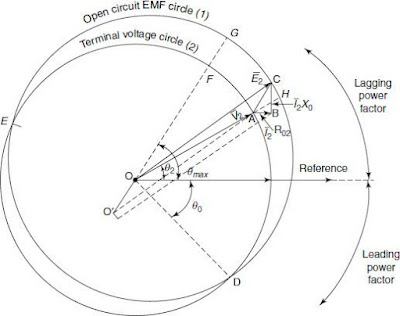WAZIPOINT Engineering Science & Technology: Kapp Regulation Diagram of a Transformer and Its Uses

## Saturday, February 12, 2022

### Kapp Regulation Diagram of a Transformer and Its Uses

Kapp regulation diagram (or in short Kapp diagram) - a graphical method of determining the voltage regulation in a transformer caused by changes in load and power factor.

The Kapp diagram is helpful in finding the voltage reduction or increase (voltage regulation).

Kapp had designed a diagram shown in the Figure below to determine the regulation at any power factor. The description of the construction of the diagram is shown below:Figure: Kapp’s Diagram

Load current (I2) is taken as a reference phasor. OA representing V2 is drawn at angle θ2 with I2. AB represents I2R02 drawn parallel to I2, whereas BC represents I2X02 drawn perpendicular to AB, i.e., I2. Here OC represents secondary emf (0V2 = E2) at no-load. Circle 1 known as often circuit EMF circle is drawn with O as centre and OC as radius.
The line OO is drawn parallel to AC representing I2Z02. With O as a centre and OA as a radius, circle 2 known as terminal voltage circle is drawn, which intersects with circle 1 at the points D and E.

The region above and below the reference line represents the lagging and leading power factors region, respectively.

Point D is the point corresponding to zero regulation. The intercept FG gives the maximum regulation, which is drawn through O and drawn parallel to AC.

The regulation at any power factor angle θ is obtained by extending OA to meet the outer circle at H. The regulation at the required power factor cosθ is represented by AH, which is the intercept between the two circles.